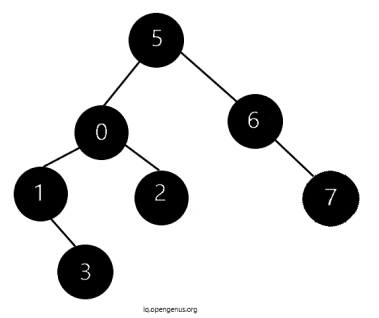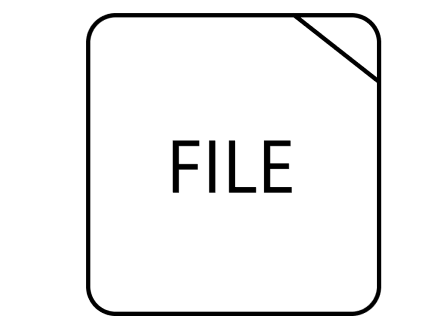×

Search anything:

# Serialization and Deserialization of Binary Tree

#### Problems on Binary Tree AlgorithmsWe have presented the process of Serialization and Deserialization of Binary Tree where we convert a Binary Tree into a compress form which can be saved in a file and retrieved back.

Serialization is the process of converting the object into bits. This makes it possible to store it in a memory buffer or a file. After serialisation, the bits can be transmitted via signals over computers and networks. On the other hand, Deserialization is the reverse of Serialisation. It converts those bits back to the binary tree. When we serialize a binary tree, one thing must be kept in mind to maintain its correct structure.

Serialization of the array is one of the basic operation that can be done.
To serialise an array string, we can apppend the length of string and then some delimeter or character. The length will help us deserialize it in future for removal of the delimeter or any character.

In deserialization of array, we will get the length inserted at starting of string then after the length from second letter onwards, traverse the letters mentioned in length. When we encounter a delimeter return the stored string and repeat the same process.

## Example of Serialization of Array:

``````//Function to serialize a string array
string arrayserialize(string s[], int l)
{
string t = "";
for (int i=0; i<l; i++)
{
int l = s[i].length();
t.push_back('0' + l);
t = t + "-" + s[i];
}
return t;
}
``````

In the above code snippet, we declare an empty string t. Then using for loop we add the length of string to "l" and push that value to string "t". Then, we add delimeter or character (here "-") and the string to temp.

So if the initial string was Opengenus, it would become 9-Opengenus.

## Example of Deserialization of Array:

``````void deserializearray(string s, string d[], int l)
{
int length, position=0;
string t = "";
int i = 0;
while(position>-1)
{
position = s.find("-", position+1);
if(position>0)
{
length = s[position-1] - 48;
t.append(s, position+1, length);
d[i++] = t;
t = "";
}
}
}
``````

We start by initialising position with 0 and declaring length variable and an empty string t. Then we use while loop to iterate from the element next to "-" in array. If the position is returned greater than 0 then we store the length of string that lies on previous index of "-". We subtracted 48 because '6' has the int value 54
if we write '6'-'0' it evaluates to 54-48, or the int 6.
Now, we append "t" with "s" from position + 1 till length of string. Then, store the string t in d array. Now, initialise the string t as empty for next iteration.

This can be implemented in variety of ways. In this article we will explore our recursive approach to tackle this problem.

## In case of Binary Tree:

Below diagram represents both conditions:

1. Convert the binary tree to bits to store in a file
2. Convert the bits from the file back to binary tree in same order.• If the given tree is Binary Search Tree then we can use preorder and postorder traversals to store it. For a general Binary Tree we can use either of preorder or postorder traversal.
• In case of Binary Tree is complete then level order traversal will be sufficient as all levels of the tree will be completely filled.
• If given tree is Binary Full Tree then every node will have either 0 or 2 children. Hence, preorder traversal will do along with storing a bit with every node to show if its internal or leaf node.

Consider the definition of the binary tree node as follows:

``````struct node{
int val;
node*left;
node*right;
}
``````

We can use preorder traversal and mark Null pointers:

For Serialization:

``````// Encodes a tree to a single string.
string serialize(node* root) {
stringstream ss;
Helper(ss, root);
return ss.str();
}
void Helper(stringstream& ss, node* cur) {
if (!cur) {
ss << "# ";
return;
}
ss << cur->val << " ";
Helper(ss, cur->left);
Helper(ss, cur->right);
}
``````

For Deserialization:

`````` // Decodes your encoded data to tree.
node* deserialize(string data) {
stringstream ss(data);
node* root = nullptr;
Helper(ss, root);
return root;
}
void Helper(stringstream& ss, node* &cur) {
string Node;
ss >> Node;
if (Node == "" || Node == "#") {
cur = nullptr;
return;
}
stringstream sss(Node);
int data;
sss >> data;
cur = new node();
cur->val = data;
cur->left = cur->right = nullptr;
Helper(ss, cur->left);
Helper(ss, cur->right);
}

``````
• In above approaches, we used the preorder traversal and marked the null as "#" after converting the tree to single string.

We can also have other approaches like Recursive Approach:

## Recurisve Approach:

### Serialization Function

We can implement the serialize function as follows:

``````string emp = "X";
void serializeRecursive(node* root, ostringstream &os) {
if (root == nullptr)  {
os << emp << " ";
return;
}
os << root->val;
os << " ";
serialize(root->left, os);
serialize(root->right, os);
}

//Encoding tree to a single string
string serialize(node* root) {
ostringstream oss;
serializeRecursive(root, oss);
return oss.str();
}
``````

## Explaination:

• Firstly, we initialise a string emp with "X".
• Then, we have two functions, serialize and serializeRecursive.
• The serialize function calls the serializeRecursive function iteratively.
• Inside the serialize function, we create an output stream "oss".
• Then we call the serializeRecursive function with root and oss as arguments.

Inside serializeRecursive function:

• The base condition is if the root is null, the output string will emp, having value "X" to denote null node.
• If the node is not null then we insert the value of root into output string and empty string for the space between two consecutive node values.
• Then, we recursively call the serializeRecurive function for traversing all the nodes of the left subtree.
• Similarly, then we recursively call the serializeRecurive function for traversing all the nodes of the right subtree.
• Once, the whole recurisve process is over, we return back to our Serialize function. Now, we return the output string "oss" as a string.

### Deserialization Function

We can implement the deserialise function as follows:

``````node * deserializeRecursive(istringstream &is) {
string val;
is >> val;
if (val == emp) {
return nullptr;
}
node *root = new node(stoi(val));
root->left = deserialize(is);
root->right = deserialize(is);
return root;
}

// Decoding your encoded data to tree
node* deserialize(string data) {
istringstream is(data);
return deserializeRecursive(is);
}
``````

## Explaination:

• Firstly, we have two functions deserialize and deserializeRecursive.
• Then, we have istringstream is(data), we pass the data in the input string stream to handle the input strings.
• Then we return deserialization function by passing "is" that contains the data of input string stream.

Inside the deserializeRecurisve function:

• Firstly, initialise the string val. Then, put it in input stream.
• Whenever, the val is equivalent to emp ( that is "X", representing the null node), we return the nullptr. It is the base condition.
• If we do not have our base condition as true then the recursive function allocates the memory for a new node.
• Similarly, we recursively iterate and reconstruct our binary tree by initialising values to our nodes. First, we call the function for left subtree and then for right subtree.
• At the end we return out root.

This way we can serialize and deserialize our binary tree.
Happy Coding!#### Srishti Guleria

Srishti Guleria has been a Technical Author, Software Developer, Intern at OpenGenus and a Computer Science student at Rajasthan Technical University, Kota

Serialization and Deserialization of Binary Tree